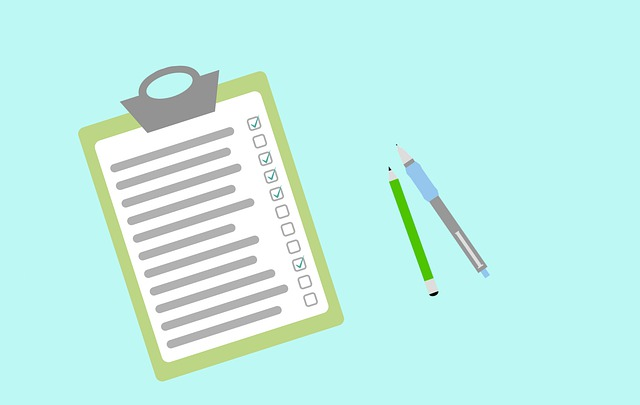# What’s tested in O Level Additional Mathematics (syllabus 4049)Do you know what’s tested in O Level Additional Mathematics?

Well, the easiest way to find out is to look at your textbook. The latest Additional Mathematics textbook should contain all the topics tested in O Levels.

Another way is to look at the official syllabus, which you can find here, from the Singapore Examinations and Academic Board (SEAB). The entire syllabus can be categorized into 3 big categories:

• Algebra
• Geometry and Trigonometry
• Calculus

Under Algebra, students are tested on:

• Equations and Inequalities
• Polynomials and Partial Fractions
• Binomial Expansions
• Surds, Exponential and Logarithmic Functions

Under Geometry and Trigonometry, students are tested on :

• Trigonometric functions and trigonometric identities and equations
• Coordinate geometry in 2 dimensions
• Coordinate geometry of circles
• Linear law (transformation of non-linear relationship to linear form)
• Proofs in Plane Geometry

Under Calculus, students are tested on :

• Differentiation and its application
• Integration and its application
• Application of differentiation and integration to problems involving displacement, velocity and acceleration of a particle moving in a straight line.

## Learn O Level A Math on-demand

Want to learn O Level A Math on-demand? Check out our course here.

error: Content is protected !!